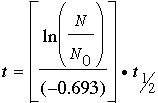SEARCH HOMEMath Central Quandaries & QueriesQuestion from Malachi, a student: I am doing carbon 14 dating and i figured out the rule for taking the percent of the original C-14 to figure out how old it is. how do I work the equation the other way around to find the percent remaining, from how long ago the objects organic mater diedMalachi,

From your previous question I know you are looking at
http://mathcentral.uregina.ca/beyond/articles/ExpDecay/Carbon14.html.

The formula in this document is. To solve for N first multiply both sides by -0.693 and then divide each side by t1/2. The result is

-0.693 t/t1/2 = ln(N/N0).

To simplify the notation let K = -0.693 t/t1/2 so that the equation becomes

K = ln(N/N0).

Now apply the exponential function to each side and you have

eK= N/N0

or

N = N0 eK.

HarleyMath Central is supported by the University of Regina and The Pacific Institute for the Mathematical Sciences.